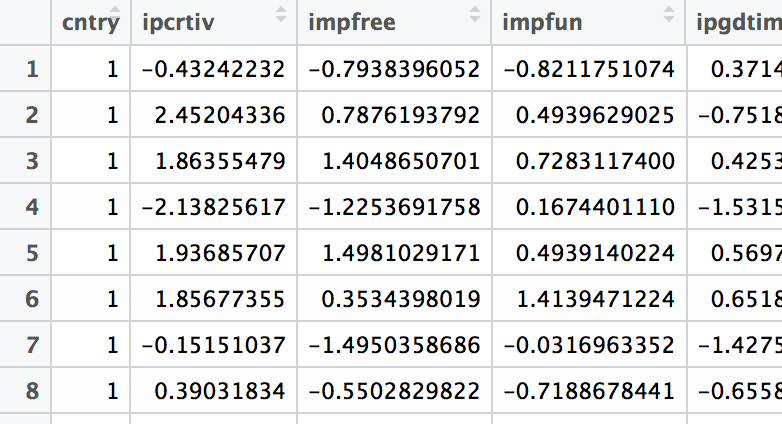## Measurement invariance explorer

First line of data file should contain variable names. First variable should be group ID, all the others - indicators. Data type: csv, space/tab delimited.

# MIE - Measurement invariance explorer

To run, type in the RStudio console: `shiny::runGitHub("MIE", "maksimrudnev")`

Invariance explorer helps to find groups that might demonstrate measurement invariance of latent factors. Instead of repeatedly running the models to test invariance, one may plot the group measures and visually select the ones that are closer to each other.

• User may interactively include and exlude groups that look different (by clicking them), and the tool will automatically refit the models.
• To facilitate combination of groups, they are also clustered.

### Covariance-based approach

Usually, assessment of measurement invariance is based on a measurement model (which is assumed to be a true model). However, one may employ completely exploratory approach, using covariances between observed variables to find groups with the most similar structures. This is the default (and the quickest) option in the `Measurement invariance explorer`. Of course, at some point one has to specify a measurement model, but it may be done after finding a set of groups with similar covariance structures.

If, or when, a measurement model is given, one can use it in `Measurement invariance explorer` to find sets of groups that are closer to each other using either model parameters or model fit indices.

### Parameter-based approach

First, one has to specify measurement model using lavaan syntax. When you add a code, `Measurement invariance explorer` will automatically compute a common measurement invariance tests based on `semTools` package. Please, insert only ready-to-use model code, as computation may take a long time. `Measurement invariance explorer` computes configural model to compare factor loadings and metric model (with fixed factor loadings) to compare intercepts. It is recommended first be sure that you found a set of groups that have similar loadings before comparing intercepts.

### Fit indices-based

When using fit indices as a measure of group proximity, `Measurement invariance explorer` computes for each pair of groups either configural and metric models , or metric and scalar models, and plots the groups based on difference between less and more restricted models, as it was suggested by Chen, 2007. Chen also suggested a threshold of .01. For convenience, a rectangle .01x.01 is added to the plot. If all the groups are inside this rectangle, it is very likely that measurement invariance holds for these groups. When using this approach please pay attention to the actual fit indices, as the implied model is assumed to be true.

### Cautions

• As follows from its name, `Measurement invariance explorer` is exploratory. The plots are based basically on averaging a bunch of information, so it doesn't guarantee that closer groups have measurement invariance.
• When using implied models, pay attention to the fit indices themselves, not only to their differences. Model in general should fit data well enough.
• The use of some fit indices in plots based on multidimensional scaling and in cluster analysis might violate assumptions, as they have not been proven to have a normal distribution / sphericity.
• With big datasets and complex models lavaan could take a considerable amount of time to get computations done. Be patient.
• One feature to be implemented is survey weights.

### Input data file structure

First column should include a group identifier, all the other columns should include indicators. Covariances are computed for all the indicators. When a model is implied, the data file might include any number of variables. However, it is recommended to keep file shorter to enhance a computation time. For example:Here's a sample code to create data for the app in R from the data.frame:

``````write.csv(mydata[,c("country", "v1", "v2", "v3", "v4", "v5", "v6")], "fileforMIE.csv", row.names = F)
``````

### Main uses

This app is very useful when there are either clusters of groups or outlier groups (in terms of implied model/correlations). The app is not very suitable for finding model misspecifications.

### Sister methods - mixture

For the more sophisticated model-based clustering see https://ppw.kuleuven.be/okp/_pdf/DeRoover2017MSFAF.pdf However this method is limited to differences in factor loadings only, does not provide information on specific between-group distances, and is heavly dependent on a single model fit index (BIC). In practive this method is available only with proprietary software.

## Credits

• Version 0.3 (alpha). Feb-10-2019
• Created by Maksim Rudnev, using Zsofia Ignacz's idea.
• Behind all multiple groups factor models stands `lavaan` package.
• Plots are built on multidimensional scaling algorithm in `cmdscale`. Inputs are Eucledean distances for covariances, correlations, and parameters, and raw increments in fit indices for the increment options.
• Clustering is `kmeans`, it is added for convienience only in order to get more dimensions to the two dimensional plot.
• The whole thing is based on ShinyApps.io

``````Rudnev M. (2018) Measurement Invariance Explorer. Retrieved from: https://github.com/MaksimRudnev/MIE